### Create an Account

Home / Questions / The following series n 2n=1 In n=1 is convergent. n + 1 Select one: O True False The volum...

# The following series n 2n=1 In n=1 is convergent. n + 1 Select one: O True False The volume of the solid generated by revolving the region bounded by 2 y = x + 1, x = 2 and the y - axis about the x -

The following series n 2n=1 In n=1 is convergent. n + 1 Select one: O True False
The volume of the solid generated by revolving the region bounded by 2 y = x + 1, x = 2 and the y - axis about the x - axis is given by : 2 (x²+ + 1)?dx Select one: True False
The partial fraction decomposition of X + 1 A Bx + C is (x - 1)2(x2 + 2) (x - 1) x²+2 + Select one: True O False
The following series n = 1 Inn nen is divergent Select one: O True O False
1 Ş 1 ex-e- dx converges 1 x Select one: True False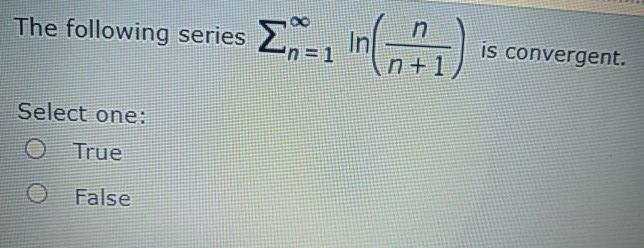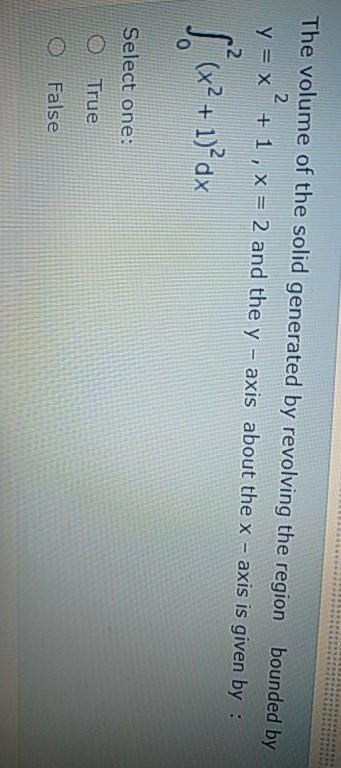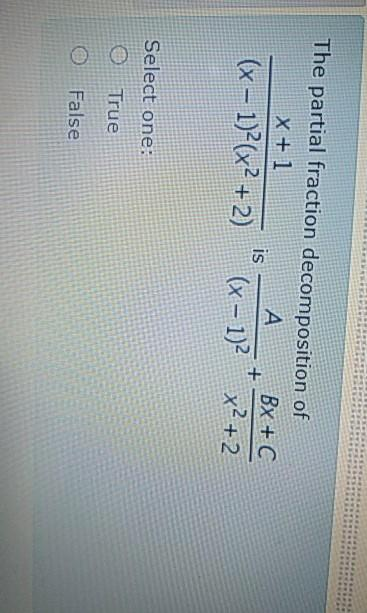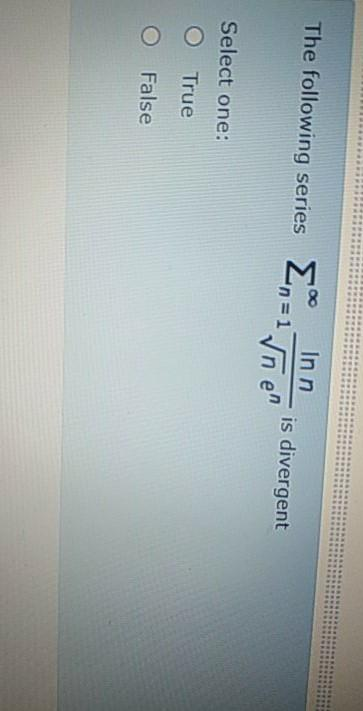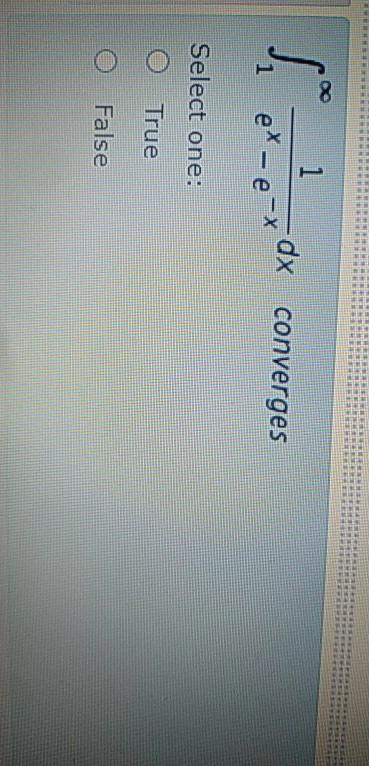Apr 24 2021 View more View LessSubscribe To Get Solution